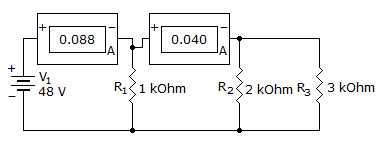# Electronics - Parallel Circuits - Discussion

Discussion Forum : Parallel Circuits - General Questions (Q.No. 15)
15.
The current through R1 will be:128 mA
88 mA
48 mA
2.2 mA
Explanation:
No answer description is available. Let's discuss.
Discussion:
17 comments Page 1 of 2.

Sushil said:   5 years ago
Thanks @Seshendra.

Vineeth K said:   6 years ago

The total current I eff is V/Reff.
Reff is equivalent resistances of r1 r2 and r3 which is 6/11.

So I=48/6 * 11= 88mA.

88mA is what comes from the source which gets divided into two parts, I1 into resistor r1 and I2 into resistor r2.

Which is given in the diagram through ammeter reading.

V is 48v across all the resistors as the voltage does not get divided in parallel connections.

So, performing I=v/r for each branch r1 r2 and r3 we get a total of 88mA with current through r1 as 48mA, r2 as 24mA and r3 as 16mA.

Armandwish said:   8 years ago
According to KCL:

I = I1+I2....I1 = I-I2.

Azhagusurya said:   8 years ago
Consider th first node:

Ii = I1+I2.
Ii = 0.088.
& I2 = 0.040.

So I1 = Ii-I2 = 0.088-0.040.

I1 = 0.048 in mA.
I1 = 48mA.

PRASANNA KUMAR said:   9 years ago
Here one simple formula is there,

In parallel circuit I = I1+I2.

IN THE CKT SHOWN ONE CURRENT IS LEAVING(0.088A) AND OTHER ENTERING(0.040A) THEN,

I+0.040 = 0.088.
I = 0.088-0.040.
= 88*10^-3 - 40*10^-3 = 48*10^-3 = 48mA.

Kamal said:   9 years ago
@Harish Kumar is wrong because the total resistance calculation is R1.R2.R3/R1R2+R2R3+R3R1.

Harish kumar said:   9 years ago
We know voltage across parallel circuits are same.
Given that V = 48v.

By ohm's law,
I=V/R.........(1).

Total resistance R1.R2.R3/R1+R2+R3.

= 1.2.3/1+2+3.

= 1 Ohm.

Substitute R value in 1,

I = 48mA.

Shiva said:   9 years ago
I = 0.088.
I1 = ?
I2 = 0.040.

Since I = I1+I2 (Kirchhoff current law).

I1 = I-I2 = 0.088 - 0.040 = 0.048 = 48 x 10^-3 = 48mA.